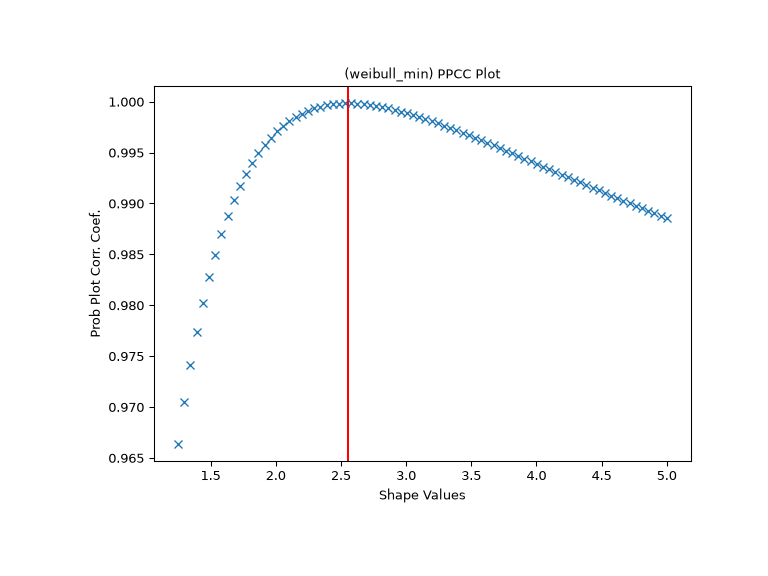# scipy.stats.ppcc_max¶

scipy.stats.ppcc_max(x, brack=(0.0, 1.0), dist='tukeylambda')[source]

Calculate the shape parameter that maximizes the PPCC.

The probability plot correlation coefficient (PPCC) plot can be used to determine the optimal shape parameter for a one-parameter family of distributions. `ppcc_max` returns the shape parameter that would maximize the probability plot correlation coefficient for the given data to a one-parameter family of distributions.

Parameters
xarray_like

Input array.

bracktuple, optional

Triple (a,b,c) where (a<b<c). If bracket consists of two numbers (a, c) then they are assumed to be a starting interval for a downhill bracket search (see `scipy.optimize.brent`).

diststr or stats.distributions instance, optional

Distribution or distribution function name. Objects that look enough like a stats.distributions instance (i.e. they have a `ppf` method) are also accepted. The default is `'tukeylambda'`.

Returns
shape_valuefloat

The shape parameter at which the probability plot correlation coefficient reaches its max value.

Notes

The brack keyword serves as a starting point which is useful in corner cases. One can use a plot to obtain a rough visual estimate of the location for the maximum to start the search near it.

References

1

J.J. Filliben, “The Probability Plot Correlation Coefficient Test for Normality”, Technometrics, Vol. 17, pp. 111-117, 1975.

2

Engineering Statistics Handbook, NIST/SEMATEC, https://www.itl.nist.gov/div898/handbook/eda/section3/ppccplot.htm

Examples

First we generate some random data from a Weibull distribution with shape parameter 2.5:

```>>> from scipy import stats
>>> import matplotlib.pyplot as plt
>>> rng = np.random.default_rng()
>>> c = 2.5
>>> x = stats.weibull_min.rvs(c, scale=4, size=2000, random_state=rng)
```

Generate the PPCC plot for this data with the Weibull distribution.

```>>> fig, ax = plt.subplots(figsize=(8, 6))
>>> res = stats.ppcc_plot(x, c/2, 2*c, dist='weibull_min', plot=ax)
```

We calculate the value where the shape should reach its maximum and a red line is drawn there. The line should coincide with the highest point in the PPCC graph.

```>>> cmax = stats.ppcc_max(x, brack=(c/2, 2*c), dist='weibull_min')
>>> ax.axvline(cmax, color='r')
>>> plt.show()
```